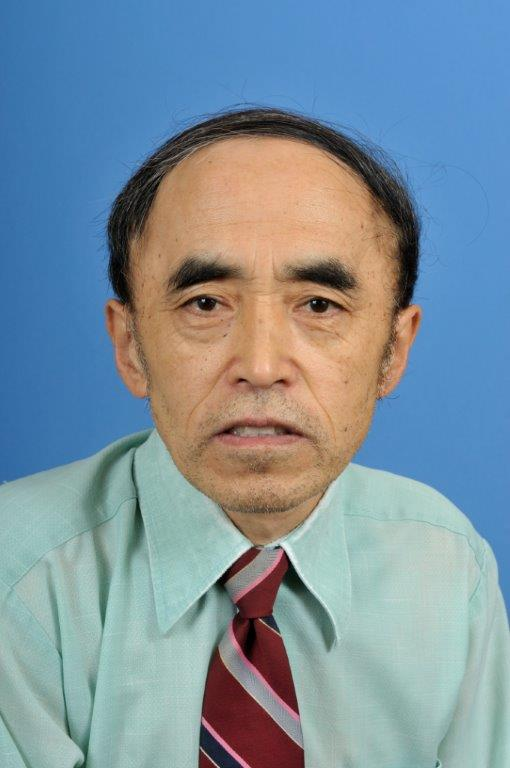# Dr. Bo ZhangProfessor in Mathematics

Office: Science & Technology Building 221

Phone: (910) 672-1786

Email:  bzhang@uncfsu.edu

Personal Homepage:  http://bzhang.fsufaculty.uncfsu.edu

BIOGRAPHY

Dr. Bo Zhang received his Ph.D. in mathematics in 1991 from Southern Illinois University at Carbondale. Currently, He is a Professor of Mathematics in the Department of Mathematics and Computer Science at Fayetteville State University. He has been serving as an Associate Editor of the "Journal of Qualitative theory of Differential Equations" since 1998, and has published more seventy research papers in journals and conference proceedings.

TEACHING

Math 121-Introduction to College Algebra
Math 123-College Algebra
Math 129-PreCalculus I
Math 130-PreCalculus II
Math 131-Algebra and Trigonometry
Math 140-Applied Calculus
Math 142-Calculus with Analytical Geometry I
Math 241-Calculus with Analytic Geometry II
Math 242-Calculus with Analytic Geometry III
Math 251-Linear Algebra
Math 320-Difference Equations
Math 331-Differential Equations I
Math 431-Differential Equations II
Math 461-Theory of Real variables
Math 507-Linear Algebra I
Math 521-Real Analysis I
Math 571-Ordinary Differential Equations
Math 611-Linear Algebra II
Math 621-Real Analysis II
Math 671-Partial Differential equations

RESEARCH INTERESTS

Qualitative theory of differential equations and dynamical systems
Stability and periodic solutions of ordinary and functional differential equations
Fixed point theory and population dynamics
Stability and Liapunov theory for fractional dynamical systems.

SELECTED PUBLICATIONS

T. A. Buton and Bo Zhang, On the reduction of Krasnoselskii's theorem to Schauder's  theorem, Applied Mathematics and Computation 250 (2015), p.339-351
Q. Tao, H. Gao, Bo Zhang, and Z. Yao, Approximate controllability of a parabolic integrodifferential equation, Mathematical Methodss in Applied Sciences 37(2014), p.2236-2244.
Bo Zhang, Contraction mapping and stability in an integro-differential equation of nonconvolution type, Dynamic Systems and Applications 23(2014), p.665-676.
T. A. Burton and Bo Zhang, A Schauder-type Fixed Point Theorem, Journal of Mathematical Analysis and Applications 417(2014), p. 552-558.
T. A. Burton and Bo Zhang, Fixed points and fractional differential equations: Examples, Fixed point Theory 14(2013), p.313-326.
T. A. Burton and Bo Zhang, Asymptotically periodic solutions of fractional differential equations, Dynamics of Continuous, Discrete, and Impulsive Systems, Series A: Mathematical Analysis 20(2013), p. 1-21.
T. A. Burton and Bo Zhang, Fractional equations and generalizations of Schaefer's and Krasnoselskii's fixed point theorems, Nonlinear Analysis 75(2012), p. 6485-6495.
T. A. Burton and Bo Zhang, Lp-Solutions of Fractional Differential Equations, Nonlinear Studies 19(2012), p. 161-177.
T. A. Burton and Bo Zhang, Boundedness and periodic solutions on integral equations, Cubo- A Mathematical Journal 14(2012), p.55-79.
Q. Tao, H. Gao, and Bo Zhang, Approximate Controllability of a parabolic Equation with Memory, Nonlinear Analysis: Hybrid Systems 6(2012), p.839-845.
T. A. Burton and Bo Zhang, Periodic Solutions of Singular Integral Equations, Journal Nonlinear Dynamics and Systems Theory 11(2011), p. 113-123.
H. Gao, Y. Li, and Bo Zhang, A Fixed Point Theorem of Krasnoselskii-Schaefer Type and Its Applications in Control and Periodicity of Integral Equations, Fixed Point Theory 12(2011), No.1, p.91-112.
Bo Zhang, Liapunov functionals and periodicity in a system of nonlinear integral equations, Electronic Journal of Qualitative Theory of Differential equations, Spec. Ed. I, 2009 No. 32, p. 1-15.
Bo Zhang, Boundedness and Global Attractivity of solutions for a system of nonlinear integral equations, Cubo- A Mathematical Journal 11(2009), p.41-53.
Bo Zhang, Periodicity in neutral functional differential equations by direct fixed point mapping, International Journal of Qualitative Theory of Differential Equations and Applications 2(2008), p. 101-111.
Bo Zhang, Formulas of Liapunov functions for systems of linear partial differential equations, Journal of Mathematical Analysis and  Applications, 325(2007), 1442-1467.  B
o Zhang, Contraction mapping and asymptotic stability in delay differential equations, Dynamics of Continuous, Discrete and Impulsive Systems, Series A 13B(2006), 317-330.
H. Gao and Bo Zhang, Fixed points and controllability in delay systems, Fixed Point Theory and Applications, Vol.2006 (2006), Article ID 41480, p.1-14.
Bo Zhang, Necessary and sufficient conditions for stability in Volterra equations of nonconvolution type, Dynamic Systems and Applications 14(2005), 525-550.
Bo Zhang, Fixed points and stability in differential equations with variable delays, Nonlinear Analysis 63(2005), e233-e242.
Bo Zhang, Contraction mapping and stability in a delay-differential equation,  Proceedings of Dynamic Systems and Applications 4(2004), 183-190.
H. Gao, P. Lei, and Bo Zhang, A class of nonlinear degenerate  integrodifferential control systems, SIAM Journal on Control and Optimization 43(2004),  986-1010.
J. R. Graef, C. Qian, and Bo Zhang, Formulas for Liapunov functions for systems of linear difference equations, Proc. London Math. Soc. 88(2004), 185-203.
T. A. Burton and Bo Zhang, Fixed points and stability of an integral equation: Nonuniqueness, Applied Mathematics letters 17(2004), 839-846.
Bo Zhang, Periodicity in dissipative-repulsive systems of functional differential equations, 5(2003), Cubo Matematica Educacional, 5(2003), 331-349.
D. Jiang, J. Wei, and Bo Zhang, Positive periodic solutions of functional differential equations  and population models, Electronic Journal of Differential Equations, 2002(2002), No.71, 1-13.
Bo Zhang, Formulas of Liapunov functions for linear ordinary and delay differential equations, Funkcialaj Ekvacioj 44(2001), 253-278.
T. A. Burton and Bo Zhang, Uniform boundedness by averaging, Acta Mathematica Hungarica 92(2001), 263-283.
Bo Zhang, Construction of Liapunov functionals for linear Volterra integrodifferential equations and stability of delay systems, Electronic Journal of Qualitative Theory of Differential Equations, Proc. Coll. Qual. Theory Differ. Equ. 2000, No.30, p.1-17.
T. Kupper, Y. Li, and Bo Zhang, Periodic solutions for dissipative repulsive systems, Tohuku Mathematical Journal 52(2000), 321-329.
P. Eloe, M. Islam, and Bo Zhang, Uniform asymptotic stability in linear Volterra integrodifferential equations with application to delay systems, Dynamic Systems and Applications 9(2000), 331-344.
J. R. Graph, C. Qian, and Bo Zhang, Asymptotic behavior of solutions of differential equations with variable delays, Proceedings of the London Mathematical Society 81(2000), 72-92.